CycleGAN，不是什么新鲜事了，写这篇也只是做个周全，最近看论文看的要吐，写个读后感缓一缓～～

### 1. issue:

• 数据集必须是成对的。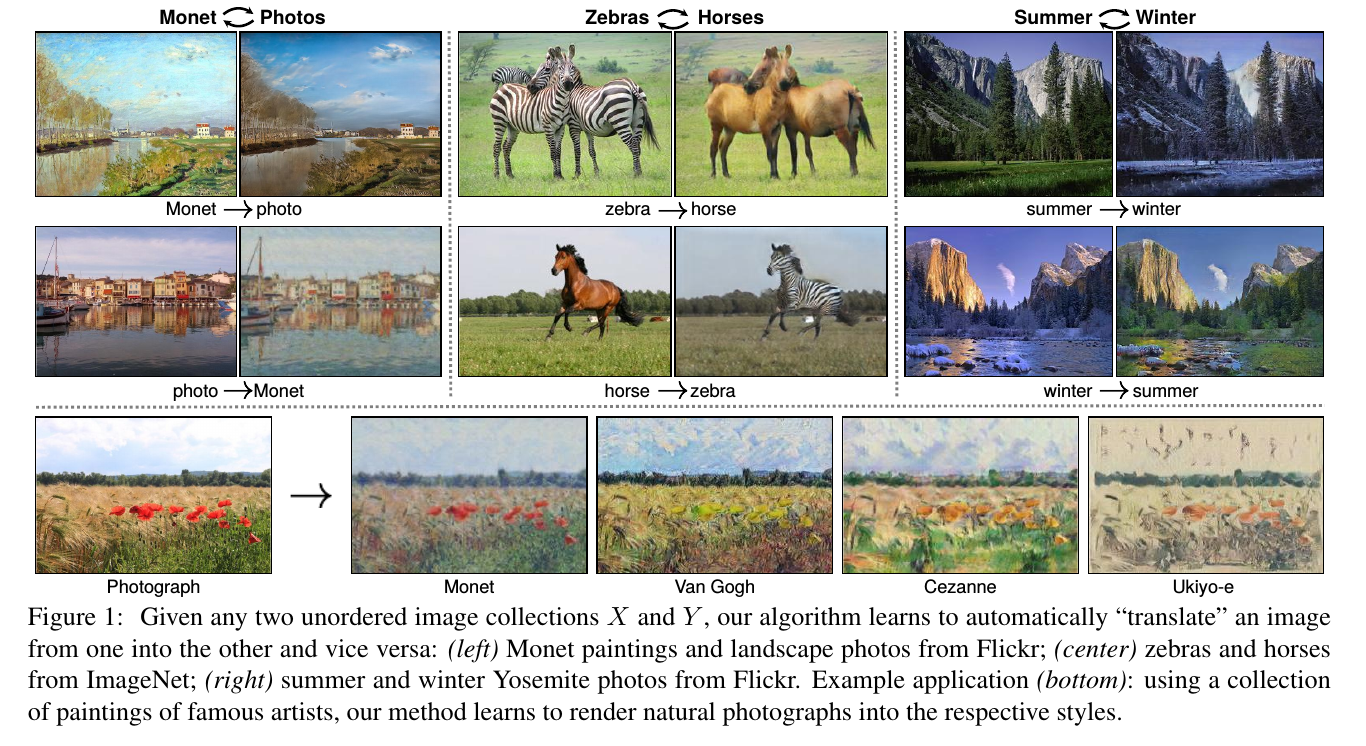)

### 2. solution:

• 汉语翻译成日语，再翻译汉语，那么得到的汉语应该跟最开始的汉语是一样的；
• 那么同理，一张图像，翻译成另一张图像，再翻译回来得到的图像，也应该和原图保持一致性。

### 3. architecture：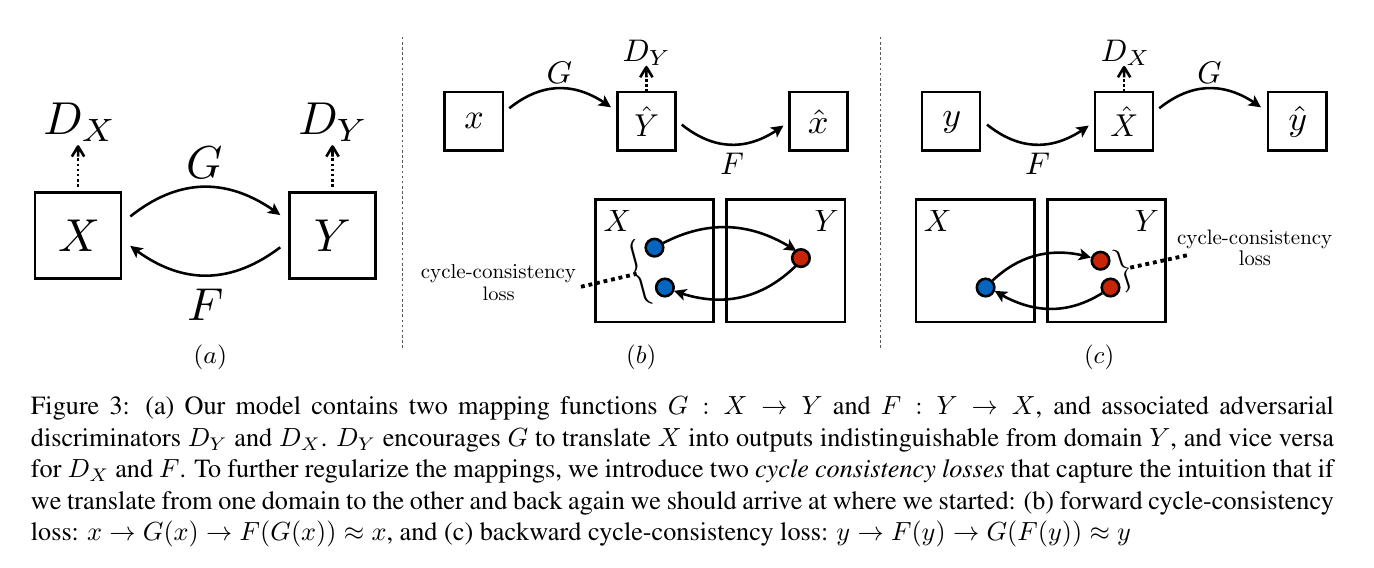• $X \to Y$;
• $Y \to X$.

$L_{GAN}(G,D_Y,X,Y)=\mathbb{E}_{y\sim P_{data}(y)}[logD_Y(y))]+\mathbb{E}_{x\sim P_{data}(x))}[log(1-D_Y(G(x))]$

$L_{GAN}(F,D_X,Y,X)=\mathbb{E}_{x\sim P_{data}(x)}[logD_X(x))]+\mathbb{E}_{y\sim P_{data}(y)}[log(1-D_X(F(y))]$

$L_{cyc}(G,F)=\mathbb{E}_{x\sim P_{data}(x)}[||F(G(x))-x||_1]+\mathbb{E}_{y\sim P_{data}(y)}[||G(F(y))-y||_1]$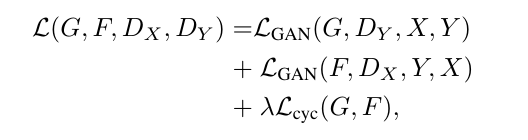### 4. implementation：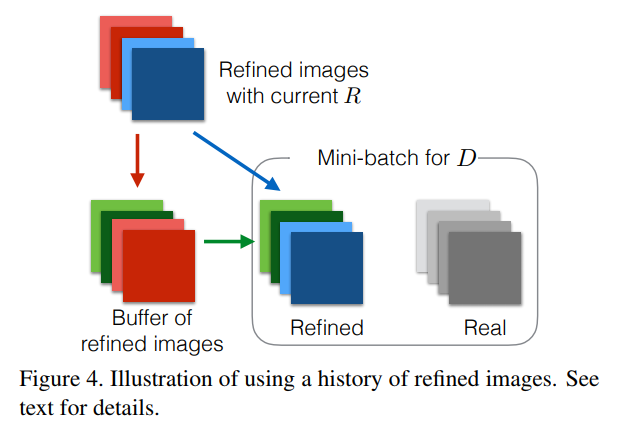### 5. results: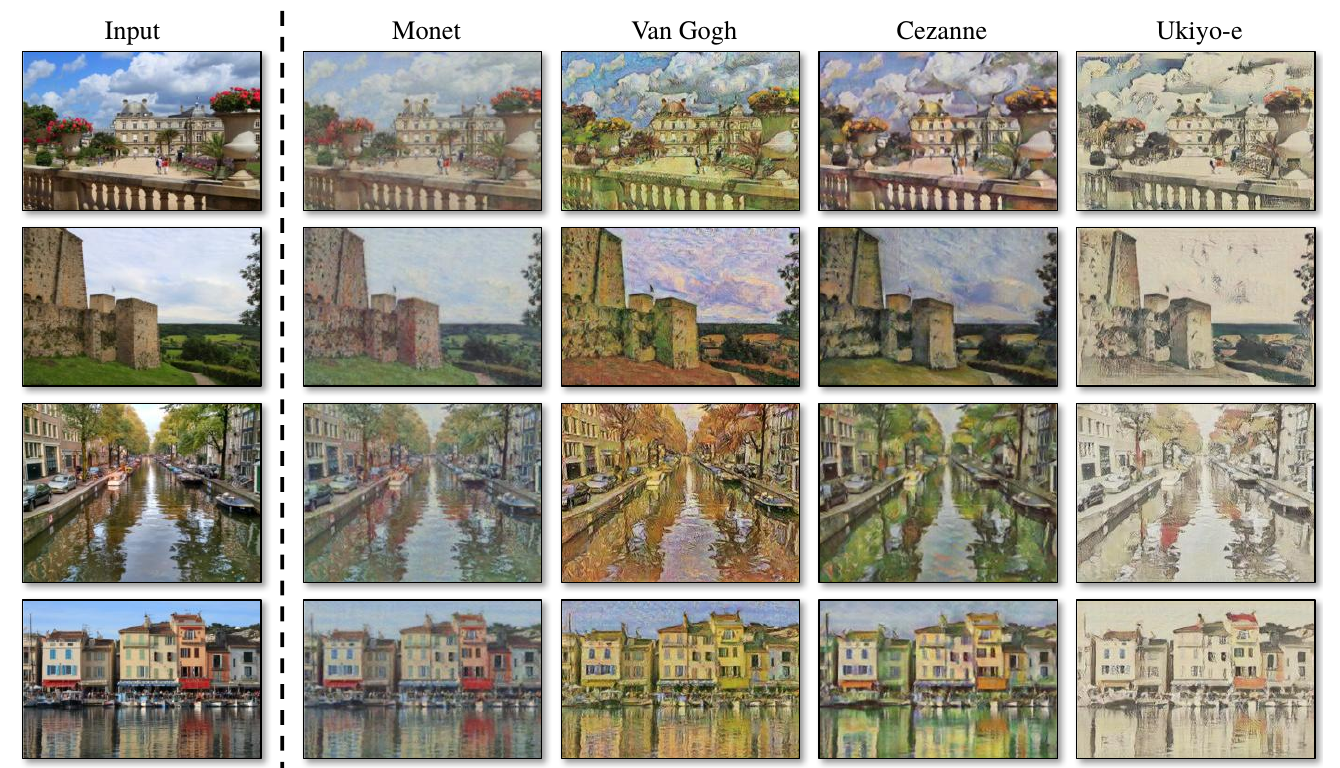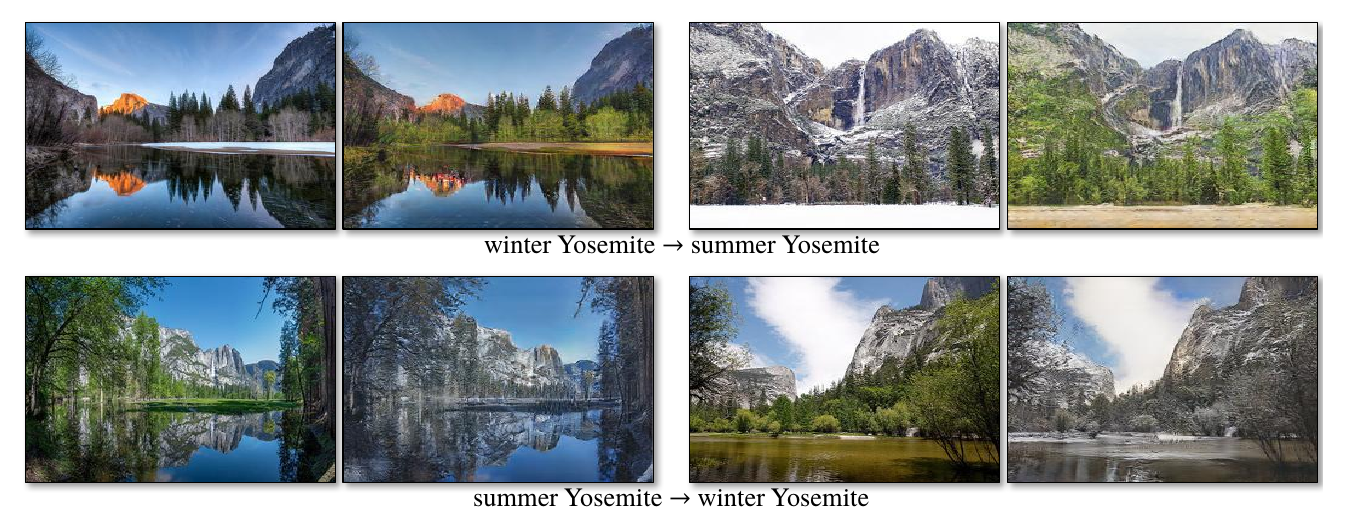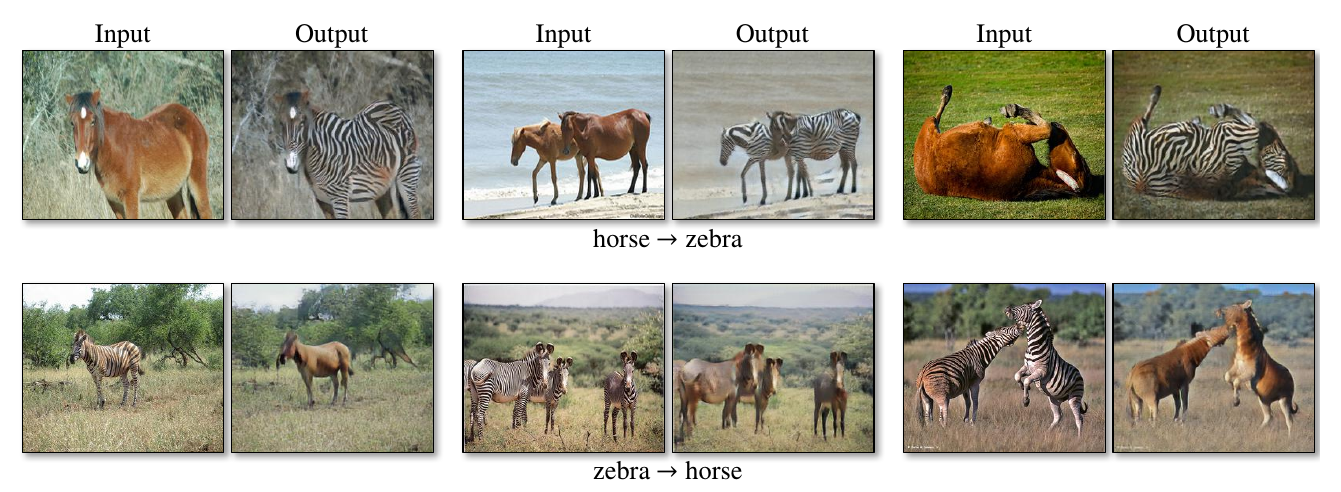regards.